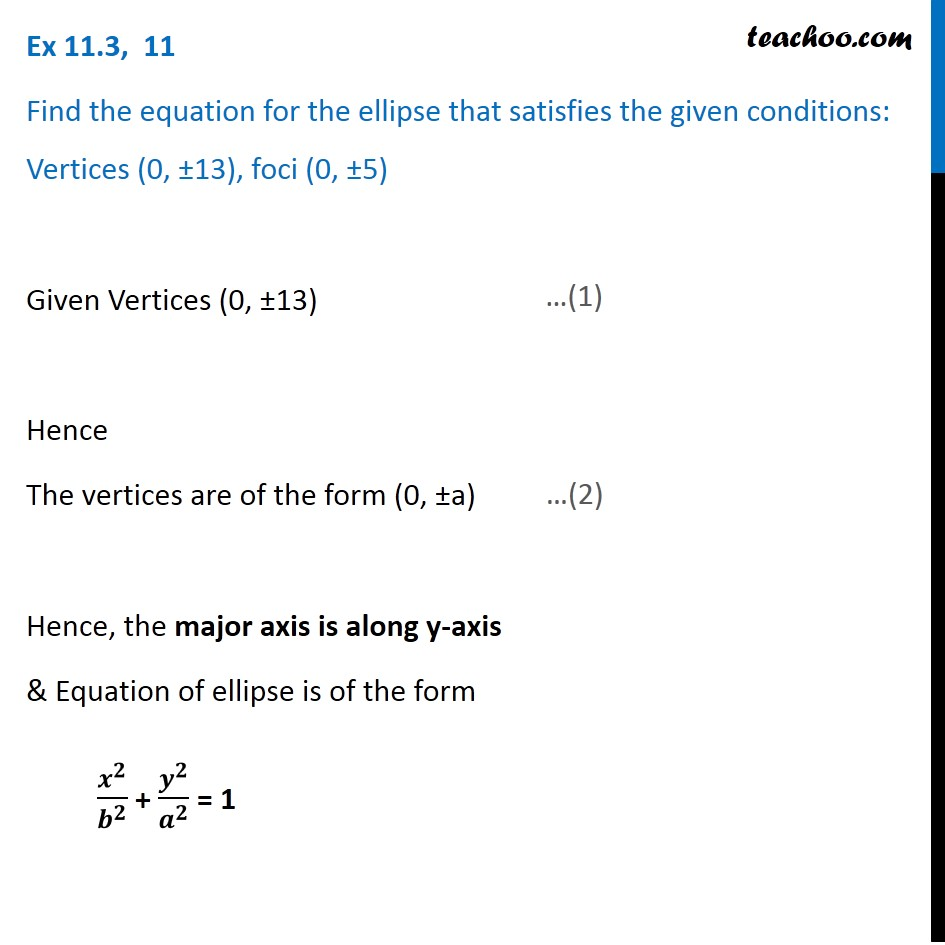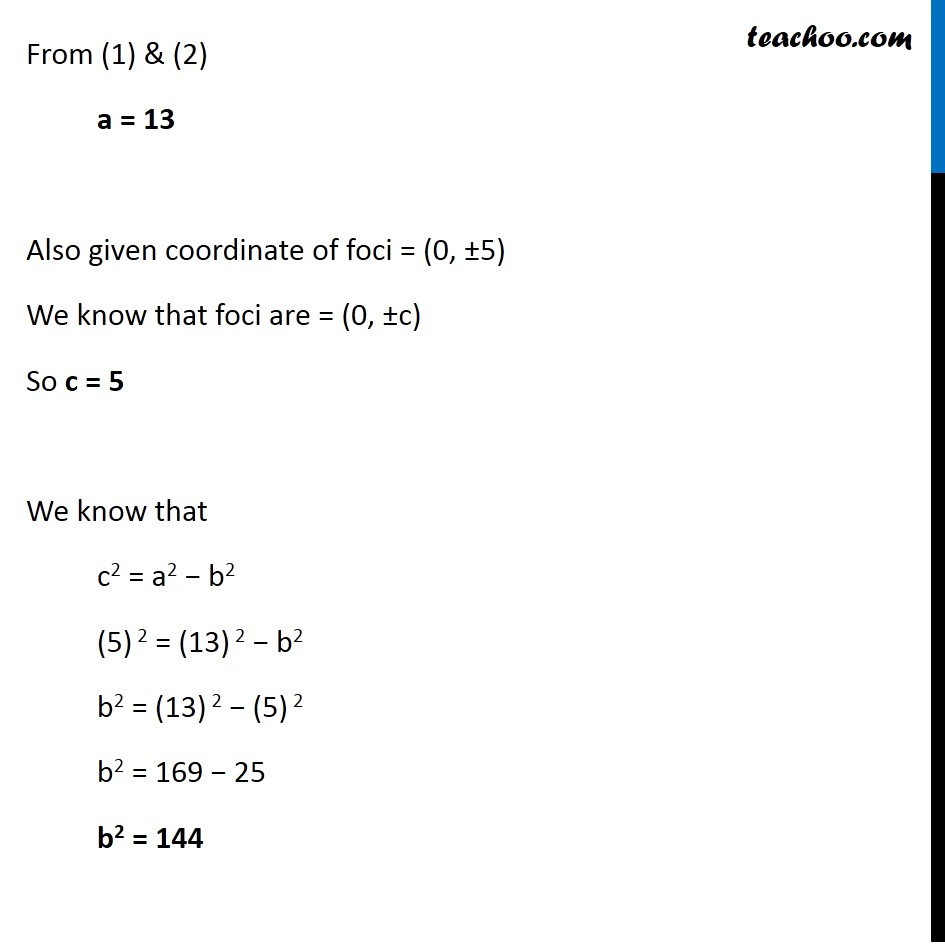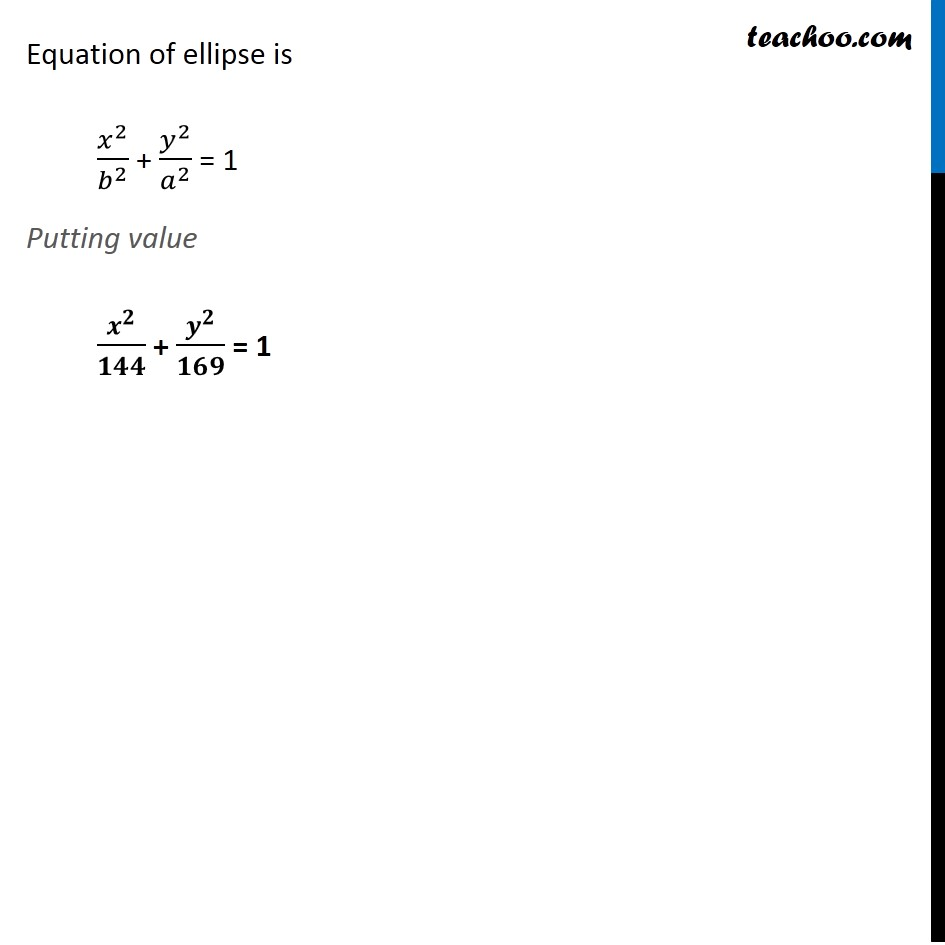1. Chapter 11 Class 11 Conic Sections (Term 2)
2. Serial order wise
3. Ex 11.3

Transcript

Ex 11.3, 11 Find the equation for the ellipse that satisfies the given conditions: Vertices (0, ±13), foci (0, ±5) Given Vertices (0, ±13) Hence The vertices are of the form (0, ±a) Hence, the major axis is along y-axis & Equation of ellipse is of the form 𝒙^𝟐/𝒃^𝟐 + 𝒚^𝟐/𝒂^𝟐 = 1 From (1) & (2) a = 13 Also given coordinate of foci = (0, ±5) We know that foci are = (0, ±c) So c = 5 We know that c2 = a2 − b2 (5) 2 = (13) 2 − b2 b2 = (13) 2 − (5) 2 b2 = 169 − 25 b2 = 144 Equation of ellipse is 𝑥^2/𝑏^2 + 𝑦^2/𝑎^2 = 1 Putting value 𝒙^𝟐/𝟏𝟒𝟒 + 𝒚^𝟐/𝟏𝟔𝟗 = 1 Equation of ellipse is 𝑥^2/𝑏^2 + 𝑦^2/𝑎^2 = 1 Putting value 𝒙^𝟐/𝟏𝟒𝟒 + 𝒚^𝟐/𝟏𝟔𝟗 = 1